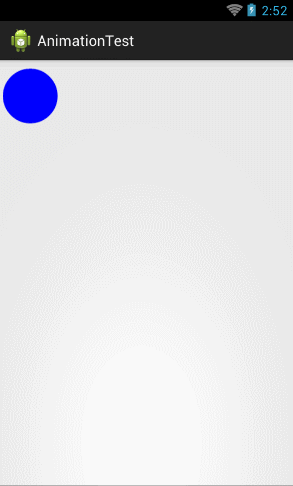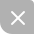# Android属性动画完全解析(中)，ValueAnimator和ObjectAnimator的高级用法

## ValueAnimator的高级用法

public class FloatEvaluator implements TypeEvaluator {
public Object evaluate(float fraction, Object startValue, Object endValue) {
float startFloat = ((Number) startValue).floatValue();
return startFloat + fraction * (((Number) endValue).floatValue() - startFloat);
}
}

public class Point {

private float x;

private float y;

public Point(float x, float y) {
this.x = x;
this.y = y;
}

public float getX() {
return x;
}

public float getY() {
return y;
}

}

Point类非常简单，只有x和y两个变量用于记录坐标的位置，并提供了构造方法来设置坐标，以及get方法来获取坐标。接下来定义PointEvaluator，如下所示：

public class PointEvaluator implements TypeEvaluator{

@Override
public Object evaluate(float fraction, Object startValue, Object endValue) {
Point startPoint = (Point) startValue;
Point endPoint = (Point) endValue;
float x = startPoint.getX() + fraction * (endPoint.getX() - startPoint.getX());
float y = startPoint.getY() + fraction * (endPoint.getY() - startPoint.getY());
Point point = new Point(x, y);
return point;
}

}

Point point1 = new Point(0, 0);
Point point2 = new Point(300, 300);
ValueAnimator anim = ValueAnimator.ofObject(new PointEvaluator(), point1, point2);
anim.setDuration(5000);
anim.start();

public class MyAnimView extends View {

public static final float RADIUS = 50f;

private Point currentPoint;

private Paint mPaint;

public MyAnimView(Context context, AttributeSet attrs) {
super(context, attrs);
mPaint = new Paint(Paint.ANTI_ALIAS_FLAG);
mPaint.setColor(Color.BLUE);
}

@Override
protected void onDraw(Canvas canvas) {
if (currentPoint == null) {
drawCircle(canvas);
startAnimation();
} else {
drawCircle(canvas);
}
}

private void drawCircle(Canvas canvas) {
float x = currentPoint.getX();
float y = currentPoint.getY();
}

private void startAnimation() {
ValueAnimator anim = ValueAnimator.ofObject(new PointEvaluator(), startPoint, endPoint);
@Override
public void onAnimationUpdate(ValueAnimator animation) {
currentPoint = (Point) animation.getAnimatedValue();
invalidate();
}
});
anim.setDuration(5000);
anim.start();
}

}

<RelativeLayout xmlns:android="http://schemas.android.com/apk/res/android"
android:layout_width="match_parent"
android:layout_height="match_parent"
>

<com.example.tony.myapplication.MyAnimView
android:layout_width="match_parent"
android:layout_height="match_parent" />

</RelativeLayout>OK！这样我们就成功实现了通过对对象进行值操作来实现动画效果的功能，这就是ValueAnimator的高级用法。

## ObjectAnimator的高级用法

ObjectAnimator的基本用法和工作原理在上一篇文章当中都已经讲解过了，相信大家都已经掌握。那么大家应该都还记得，我们在吐槽补间动画的时候有提到过，补间动画是只能实现移动、缩放、旋转和淡入淡出这四种动画操作的，功能限定死就是这些，基本上没有任何扩展性可言。比如我们想要实现对View的颜色进行动态改变，补间动画是没有办法做到的。

public class MyAnimView extends View {

...

private String color;

public String getColor() {
return color;
}

public void setColor(String color) {
this.color = color;
mPaint.setColor(Color.parseColor(color));
invalidate();
}

...

}

public class ColorEvaluator implements TypeEvaluator {

private int mCurrentRed = -1;

private int mCurrentGreen = -1;

private int mCurrentBlue = -1;

@Override
public Object evaluate(float fraction, Object startValue, Object endValue) {
String startColor = (String) startValue;
String endColor = (String) endValue;
int startRed = Integer.parseInt(startColor.substring(1, 3), 16);
int startGreen = Integer.parseInt(startColor.substring(3, 5), 16);
int startBlue = Integer.parseInt(startColor.substring(5, 7), 16);
int endRed = Integer.parseInt(endColor.substring(1, 3), 16);
int endGreen = Integer.parseInt(endColor.substring(3, 5), 16);
int endBlue = Integer.parseInt(endColor.substring(5, 7), 16);
// 初始化颜色的值
if (mCurrentRed == -1) {
mCurrentRed = startRed;
}
if (mCurrentGreen == -1) {
mCurrentGreen = startGreen;
}
if (mCurrentBlue == -1) {
mCurrentBlue = startBlue;
}
// 计算初始颜色和结束颜色之间的差值
int redDiff = Math.abs(startRed - endRed);
int greenDiff = Math.abs(startGreen - endGreen);
int blueDiff = Math.abs(startBlue - endBlue);
int colorDiff = redDiff + greenDiff + blueDiff;
if (mCurrentRed != endRed) {
mCurrentRed = getCurrentColor(startRed, endRed, colorDiff, 0,
fraction);
} else if (mCurrentGreen != endGreen) {
mCurrentGreen = getCurrentColor(startGreen, endGreen, colorDiff,
redDiff, fraction);
} else if (mCurrentBlue != endBlue) {
mCurrentBlue = getCurrentColor(startBlue, endBlue, colorDiff,
redDiff + greenDiff, fraction);
}
// 将计算出的当前颜色的值组装返回
String currentColor = "#" + getHexString(mCurrentRed)
+ getHexString(mCurrentGreen) + getHexString(mCurrentBlue);
return currentColor;
}

/**
* 根据fraction值来计算当前的颜色。
*/
private int getCurrentColor(int startColor, int endColor, int colorDiff,
int offset, float fraction) {
int currentColor;
if (startColor > endColor) {
currentColor = (int) (startColor - (fraction * colorDiff - offset));
if (currentColor < endColor) {
currentColor = endColor;
}
} else {
currentColor = (int) (startColor + (fraction * colorDiff - offset));
if (currentColor > endColor) {
currentColor = endColor;
}
}
return currentColor;
}

/**
* 将10进制颜色值转换成16进制。
*/
private String getHexString(int value) {
String hexString = Integer.toHexString(value);
if (hexString.length() == 1) {
hexString = "0" + hexString;
}
return hexString;
}

}

ObjectAnimator anim = ObjectAnimator.ofObject(myAnimView, "color", new ColorEvaluator(),
"#0000FF", "#FF0000");
anim.setDuration(5000);
anim.start();

public class MyAnimView extends View {

...

private void startAnimation() {
ValueAnimator anim = ValueAnimator.ofObject(new PointEvaluator(), startPoint, endPoint);
@Override
public void onAnimationUpdate(ValueAnimator animation) {
currentPoint = (Point) animation.getAnimatedValue();
invalidate();
}
});
ObjectAnimator anim2 = ObjectAnimator.ofObject(this, "color", new ColorEvaluator(),
"#0000FF", "#FF0000");
AnimatorSet animSet = new AnimatorSet();
animSet.play(anim).with(anim2);
animSet.setDuration(5000);
animSet.start();
}

}OK，位置动画和颜色动画非常融洽的结合到一起了，看上去效果还是相当不错的，这样我们就把ObjectAnimator的高级用法也掌握了。UP更新不错过~
06-19195
03-07582
10-131504
08-12316
04-0920万+
07-016435
12-19975
07-132822
10-09621
05-25546
01-09
07-23126
09-121875
12-0247

### “相关推荐”对你有帮助么？

•非常没帮助
•没帮助
•一般
•有帮助
•非常有帮助被折叠的  条评论 为什么被折叠?到【灌水乐园】发言guolin

¥2 ¥4 ¥6 ¥10 ¥20余额支付 (余额：-- )扫码支付获取中扫码支付点击重新获取扫码支付1.余额是钱包充值的虚拟货币，按照1:1的比例进行支付金额的抵扣。
2.余额无法直接购买下载，可以购买VIP、C币套餐、付费专栏及课程。余额充值